文本的检测、识别实战：使用 Tesseract 进行 OpenCV OCR 和文本识别

+关注继续查看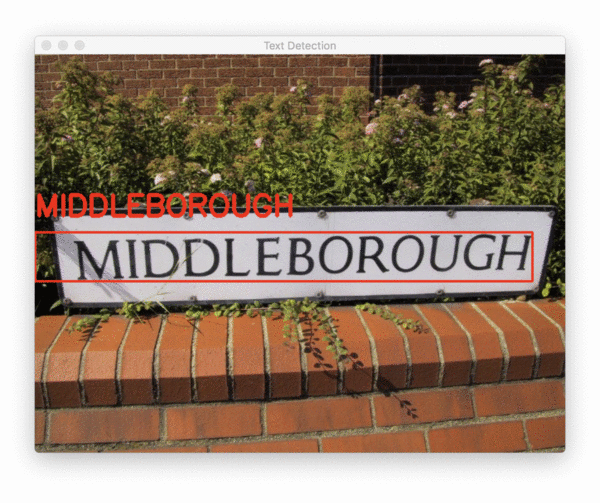lsb_release -a

1

sudo apt install tesseract-ocr

1

brew install tesseract

1

1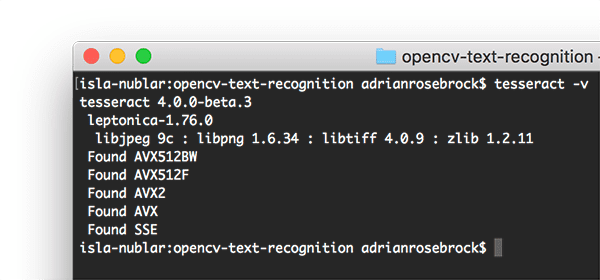tesseract -v

1

$pip install pillow$ pip install pytesseract

$pip install imutils 1 2 3 了解 OpenCV OCR 和 Tesseract 文本识别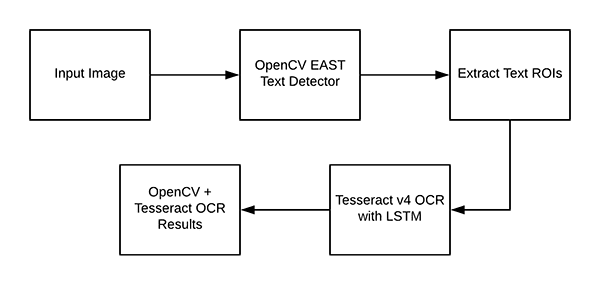现在我们已经在我们的系统上成功安装了 OpenCV 和 Tesseract，我们需要简要回顾一下我们的管道和相关命令。 首先，我们将应用 OpenCV 的 EAST 文本检测器来检测图像中文本的存在。 EAST 文本检测器将为我们提供文本 ROI 的边界框 (x, y) 坐标。 我们将提取这些 ROI 中的每一个，然后将它们传递给 Tesseract v4 的 LSTM 深度学习文本识别算法。 LSTM 的输出将为我们提供实际的 OCR 结果。 最后，我们将在输出图像上绘制 OpenCV OCR 结果。 但在我们真正开始我们的项目之前，让我们简要回顾一下 Tesseract 命令（它将被 pytesseract 库在后台调用）。 在调用 tessarct 二进制文件时，我们需要提供许多标志。三个最重要的是 -l 、 --oem 和 --psm 。 -l 标志控制输入文本的语言。我们将在此示例中使用 eng（英语），但您可以在此处查看 Tesseract 支持的所有语言。 –oem 参数或 OCR 引擎模式控制 Tesseract 使用的算法类型。 您可以通过执行以下命令查看可用的 OCR 引擎模式：$ tesseract --help-oem
OCR Engine modes:
0    Legacy engine only.
1    Neural nets LSTM engine only.
2    Legacy + LSTM engines.
3    Default, based on what is available.

tesseract --help-psm
Page segmentation modes:
0    Orientation and script detection (OSD) only.
1    Automatic page segmentation with OSD.
2    Automatic page segmentation, but no OSD, or OCR.
3    Fully automatic page segmentation, but no OSD. (Default)
4    Assume a single column of text of variable sizes.
5    Assume a single uniform block of vertically aligned text.
6    Assume a single uniform block of text.
7    Treat the image as a single text line.
8    Treat the image as a single word.
9    Treat the image as a single word in a circle.
10    Treat the image as a single character.
11    Sparse text. Find as much text as possible in no particular order.
12    Sparse text with OSD.
13    Raw line. Treat the image as a single text line,
bypassing hacks that are Tesseract-specific.

项目结构

tree --dirsfirst
.
├── images
│   ├── example_01.jpg
│   ├── example_02.jpg
│   ├── example_03.jpg
│   ├── example_04.jpg
│   └── example_05.jpg
├── frozen_east_text_detection.pb
└── text_recognition.py

images/ ：包含六个包含场景文本的测试图像的目录。 我们将对这些图像中的每一个尝试 OpenCV OCR。

frozen_east_text_detection.pb：EAST 文本检测器。 该 CNN 已针对文本检测进行了预训练，可以立即使用。

text_recognition.py ：我们的 OCR 脚本——我们将逐行查看这个脚本。 该脚本利用 EAST 文本检测器来查找图像中的文本区域，然后利用 Tesseract v4 进行识别。

# import the necessary packages
from imutils.object_detection import non_max_suppression
import numpy as np
import pytesseract
import argparse
import cv2

def decode_predictions(scores, geometry):
# grab the number of rows and columns from the scores volume, then
# initialize our set of bounding box rectangles and corresponding
# confidence scores
(numRows, numCols) = scores.shape[2:4]
rects = []
confidences = []
# loop over the number of rows
for y in range(0, numRows):
# extract the scores (probabilities), followed by the
# geometrical data used to derive potential bounding box
# coordinates that surround text
scoresData = scores[0, 0, y]
xData0 = geometry[0, 0, y]
xData1 = geometry[0, 1, y]
xData2 = geometry[0, 2, y]
xData3 = geometry[0, 3, y]
anglesData = geometry[0, 4, y]
# loop over the number of columns
for x in range(0, numCols):
# if our score does not have sufficient probability,
# ignore it
if scoresData[x] < args["min_confidence"]:
continue
# compute the offset factor as our resulting feature
# maps will be 4x smaller than the input image
(offsetX, offsetY) = (x * 4.0, y * 4.0)
# extract the rotation angle for the prediction and
# then compute the sin and cosine
angle = anglesData[x]
cos = np.cos(angle)
sin = np.sin(angle)
# use the geometry volume to derive the width and height
# of the bounding box
h = xData0[x] + xData2[x]
w = xData1[x] + xData3[x]
# compute both the starting and ending (x, y)-coordinates
# for the text prediction bounding box
endX = int(offsetX + (cos * xData1[x]) + (sin * xData2[x]))
endY = int(offsetY - (sin * xData1[x]) + (cos * xData2[x]))
startX = int(endX - w)
startY = int(endY - h)
# add the bounding box coordinates and probability score
# to our respective lists
rects.append((startX, startY, endX, endY))
confidences.append(scoresData[x])
# return a tuple of the bounding boxes and associated confidences
return (rects, confidences)

decode_predictions 函数，在 EAST 文本检测帖子中有详细解释。

# construct the argument parser and parse the arguments
ap = argparse.ArgumentParser()
help="path to input image")
help="path to input EAST text detector")
help="minimum probability required to inspect a region")
help="nearest multiple of 32 for resized width")
help="nearest multiple of 32 for resized height")
args = vars(ap.parse_args())

–image ：输入图像的路径。

–east ：预训练 EAST 文本检测器的路径。

–min-confidence ：检测到的文本区域的最小概率。

–width ：我们的图像在通过 EAST 文本检测器之前将调整到的宽度。 我们的检测器需要 32 的倍数。

–height ：与宽度相同，但用于高度。 同样，我们的检测器需要 32 的倍数来调整高度。

–padding ：添加到每个 ROI 边框的（可选）填充量。 如果您发现 OCR 结果不正确，您可以尝试使用 0.05 表示 5% 或 0.10 表示 10%（依此类推）。

# load the input image and grab the image dimensions
orig = image.copy()
(origH, origW) = image.shape[:2]
# set the new width and height and then determine the ratio in change
# for both the width and height
(newW, newH) = (args["width"], args["height"])
rW = origW / float(newW)
rH = origH / float(newH)
# resize the image and grab the new image dimensions
image = cv2.resize(image, (newW, newH))
(H, W) = image.shape[:2]

# define the two output layer names for the EAST detector model that
# we are interested in -- the first is the output probabilities and the
# second can be used to derive the bounding box coordinates of text
layerNames = [
"feature_fusion/Conv_7/Sigmoid",
"feature_fusion/concat_3"]
# load the pre-trained EAST text detector

# construct a blob from the image and then perform a forward pass of
# the model to obtain the two output layer sets
blob = cv2.dnn.blobFromImage(image, 1.0, (W, H),
(123.68, 116.78, 103.94), swapRB=True, crop=False)
net.setInput(blob)
(scores, geometry) = net.forward(layerNames)
# decode the predictions, then  apply non-maxima suppression to
# suppress weak, overlapping bounding boxes
(rects, confidences) = decode_predictions(scores, geometry)
boxes = non_max_suppression(np.array(rects), probs=confidences)

# initialize the list of results
results = []
# loop over the bounding boxes
for (startX, startY, endX, endY) in boxes:
# scale the bounding box coordinates based on the respective
# ratios
startX = int(startX * rW)
startY = int(startY * rH)
endX = int(endX * rW)
endY = int(endY * rH)
# in order to obtain a better OCR of the text we can potentially
# apply a bit of padding surrounding the bounding box -- here we
# are computing the deltas in both the x and y directions
dX = int((endX - startX) * args["padding"])
dY = int((endY - startY) * args["padding"])
# apply padding to each side of the bounding box, respectively
startX = max(0, startX - dX)
startY = max(0, startY - dY)
endX = min(origW, endX + (dX * 2))
endY = min(origH, endY + (dY * 2))
# extract the actual padded ROI
roi = orig[startY:endY, startX:endX]

• 根据先前计算的比率缩放边界框。
• 填充边界框。
• 最后，提取填充的 roi（第 144 行）。

# in order to apply Tesseract v4 to OCR text we must supply
# (1) a language, (2) an OEM flag of 4, indicating that the we
# wish to use the LSTM neural net model for OCR, and finally
# (3) an OEM value, in this case, 7 which implies that we are
# treating the ROI as a single line of text
config = ("-l eng --oem 1 --psm 7")
text = pytesseract.image_to_string(roi, config=config)
# add the bounding box coordinates and OCR'd text to the list
# of results
results.append(((startX, startY, endX, endY), text))

pytesseract 库负责处理我们调用 pytesseract.image_to_string 的第 152 行的其余部分，传递我们的 roi 和 config string 。

# sort the results bounding box coordinates from top to bottom
results = sorted(results, key=lambda r:r)
# loop over the results
for ((startX, startY, endX, endY), text) in results:
# display the text OCR'd by Tesseract
print("OCR TEXT")
print("========")
print("{}\n".format(text))
# strip out non-ASCII text so we can draw the text on the image
# using OpenCV, then draw the text and a bounding box surrounding
# the text region of the input image
text = "".join([c if ord(c) < 128 else "" for c in text]).strip()
output = orig.copy()
cv2.rectangle(output, (startX, startY), (endX, endY),
(0, 0, 255), 2)
cv2.putText(output, text, (startX, startY - 20),
cv2.FONT_HERSHEY_SIMPLEX, 1.2, (0, 0, 255), 3)
# show the output image
cv2.imshow("Text Detection", output)
cv2.waitKey(0)

OpenCV 文本识别结果

python text_recognition.py --east frozen_east_text_detection.pb \
--image images/example_01.jpg
OCR TEXT
========
OH OK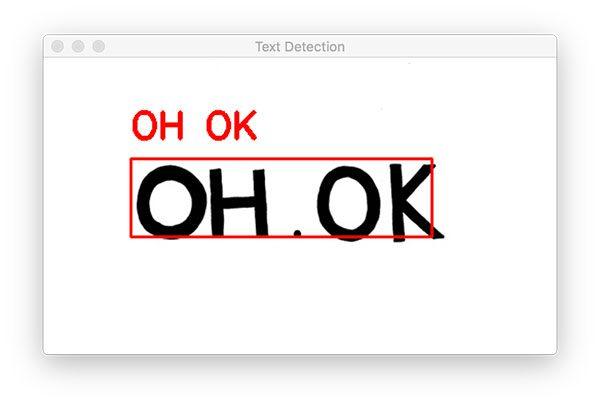python text_recognition.py --east frozen_east_text_detection.pb \
--image images/example_02.jpg
OCR TEXT
========
® MIDDLEBOROUGH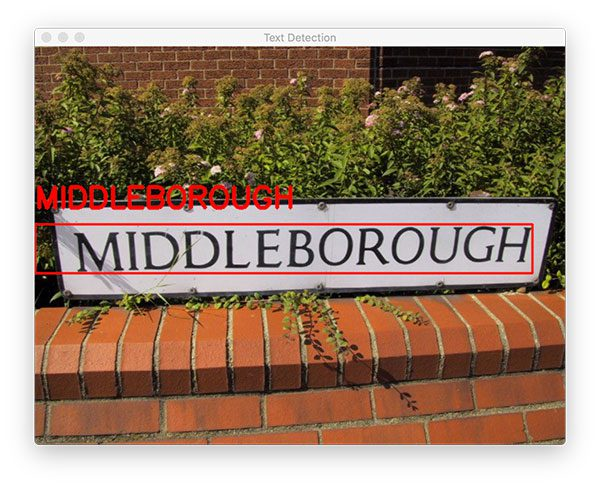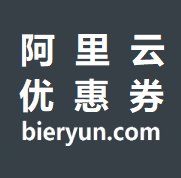1597 025 行 Python 代码实现人脸检测——OpenCV 技术教程
2338 08374 01366 0Keepalived+Nginx反向代理检测集群节点状态企业实战
1213 0js 正则之检测素数

868 0Mirantis使用Kubernetes容器化OpenStack以缓解运维挑战

1434 0《树莓派Python编程入门与实战》——2.2　使用Raspbian命令行
2306 0symbian使用HookLogger检测内存泄漏

853 0Docker实战(六)之使用Dockerfile创建镜像
Dockervile是一个文本格式的配置文件，用户可以使用Dockerfile来快速创建自定义镜像。 1.基本结构 Dockerfile由一行行命令语句组成，并且支持以#开头的注释行。 一般而言，Dockerfile分为四部分:基础镜像信息、维护者信息、镜像操作指令和容器启动时执行命令。
1343 0+关注
390

0

《2021云上架构与运维峰会演讲合集》

《零基础CSS入门教程》

《零基础HTML入门教程》